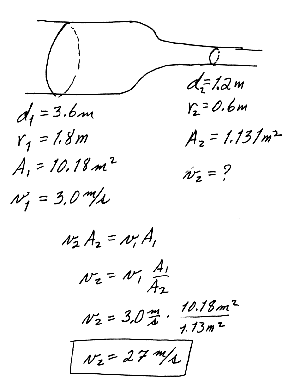## Ch 15, Fluid Mechanics

### (Yikes! Nine this time! These, too, had better be good! (and they are; that's why I got so carried away.))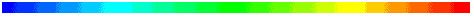Additional problems from Serway's fourth edition

(4 ed) 15.1 A king orders a gold crown having a mass of 0.5 kg. When it arrives from the metalsmith, the volume of the crown is found to be 185 cm3. Is the crown made of solid gold?

(4 ed) 15.2 Determine the absolute pressure at the bottom of a lake that is 30 m deep.

(4 ed) 15.3 A simple U-tube that is open at both ends is partially filled with water (Fig. P(4)15.3). Keroseen of density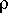k is then poured into one arm of the tube, forming a column of height hk, as shown in the diagram. What is the difference h in the heights of the two liquid surfaces?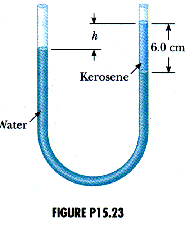(4 ed) 15.4 The rate of flow of water through a horizontal pipe is 2.00 m3/min. Determine the speed of flow at a point where the diameter of the pipe is

(a) 10.0 cm, and

(b) 5.0 cm.

(4 ed) 15.5 The Garfield Thomas water tunnel at Pennsylvania State University has a circular cross-section that constricts from a diameter of 3.6 m to the test section which is 1.2 m in diameter. If the speed of flow is 3.0 m/s in the larger-diameter pipe, determine the speed of flow in the test section.Conceptual Questions

Q15.3 When you drink a liquid through a staraw, you reduce the pressure in your mouth and let the atmospher move the liquid. Explain why this is so. Can you use a straw to sip a drink on the Moon?

In our common, ordinary surroundings, the atmosphere with its pressure is always present. Therefore if the pressure is reduced by your mouth and a straw the ordinary air pressure exerts forces on the liquid into straw where the pressure is less.

No, a straw will not function on the Moon because there is no atmosphere.

Q15.6 Does a ship ride higher in the water of an inland lake or in the ocean?

The weight of a ship is often called its "displacement" because that is the weight of the water that it displaces. It will float when it displaces a volume of water whose weight is equal to the weight of the ship -- this is the buoyant force provided by the water. Fresh water in an inland lake has a smaller density than that of sea water. Therefore, a larger volume of fresh water is necessary to provide the same weight or buoyant force. This means the ship will ride lower in an inland lake and will ride higher in the ocean.

Q15.7 Lead has a greater density than iron, and both metals are denser than water. Is the buoyant force on a lead object greater than, less than, or equal to the buoyant force on an iron object of the same volume?

The two are equal because the buoyant force depends only upon the density and volume of the liquid that is displaced.

Q15.8 The water supply for a city is often provided by reservoirs built on high ground. Water flows from the reservoir through pipes and into your home when you turn the tap on your faucet. Why is the flow of water more rapid out of a faucet on the first floor of a building than it is in an apartment on a higher floor?

The flow of water depends upon the difference in pressure between the pressure in the pipe and the air pressure outside the pipe. The air pressure is essentiall the same on the first floor or a higher floor because the density of air is small (compared to the density of water). However, the water pressure will decrease as the altitude increases. This means the difference in pressure between water pressure inside the pipe and air pressure outside the pipe will be smaller as one's altitude increases.

Q15.9 Smoke rises in a chimney faster when a breeze is blowing than wehn there is no breeze at all. Use Bernoulii's equation to explain this phenomenon.

As the speed of the air across the chimney increases, the pressure decreases. The pressure inside the house will remain constant. So a decrease in pressure at the top of the chimney means the difference in pressure between the fireplace inside the house and the top of the chimney, open to the moving air, increases and that moves the smoke more.

Q15.16 Because atmospheric pressure is about 105 N/m2 and the area of a person's chest is about 0.13 m2, the force of the atmosphere on one's chest is around 13 000 N. In view of this enormous force, why don't our bodies collapse?

It is always the net force that we need to be concerned with. Here, it would be the net pressure or the difference in pressure that we need to be concerned with. While there may be a force of 13 000 N on the outside of our chests, there is an equal 13 000 N on the inside of our chests. So the net force due to the air is zero.

Q15.17 How would you determine the density of an irregularly shaped rock?

If you submerge the rock you can measure the increase in the depth of the water and determine the volume of the water displace and, thus, the volume of the rock. You could fill a container to the very brim with water and submerge the rock. In this case the water will overflow and this amount of water that overflows is just equal to the volume of the rock. Once the volume of the rock is established, the density is just the mass of the rock divided by its volume.

Q15.22 Prairie dogs ventilate their burrows by building a mound over one entrance, which is open to a stream of air. A second entrance at ground level is open to almost stagnant air. How does this construction create an air flow through the burrow?

According to Bernoulli's Equation, flowing air exerts less pressure than still or stagnant air. Therefore, the air pressure at the mound entrance, with air flowing by it, will be less than the air pressure at the ground level entrance. This difference in air pressure will move air in at the ground level entrance and out at the mound entrance.

Clever little fellows, those prairie dogs, eh?

These same ideas are involved with air flowing through a fireplace and out a chimney as we saw in question Q15.9.

Q15.23 An unopened can of diet cola floats when placed in a tank of water whereas a can of regular cola of the same brand sinks in the tank. What do you suppose could explain this phenomenon?

Although they have the same volume, the regular cola must have a greater weight.Problems from the current (5th) edition of Serway and Beichner.

15.12 A swimming pool has dimensions 30 m x 10 m and a flat bottom. When the pool is filled to a depth of 2.0 m with fresh water, what is the total force due to the water on the bottom? On each end? On each side?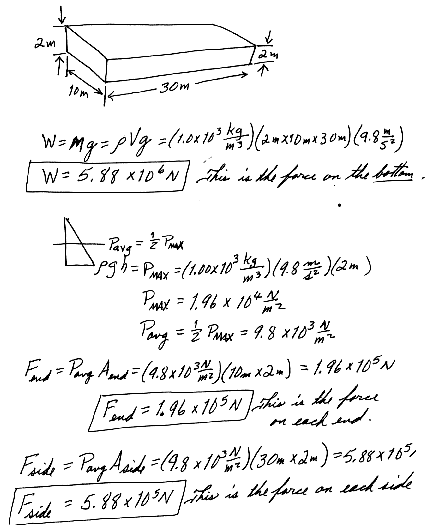15.18 Mercury is poured into a U-tube as in Figure P15.18a. The left arm of the tube has a cross-sectional area of A1 = 10.0 cm2, an dthe right arm has a cross-sectional area of A2 = 5.00 cm2. One hundred grams of water are then poured into the right arm as in Figure P15.18b.

(a) Determine the length of the water column in the right arm of the U-tube.

(b) Given that the density of mercury is 13.6 g/cm3, what distance, h, does the mercury rise in the left arm?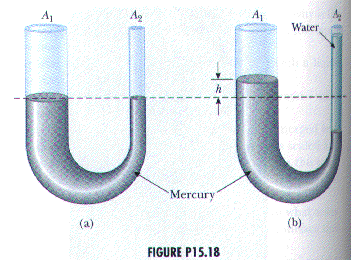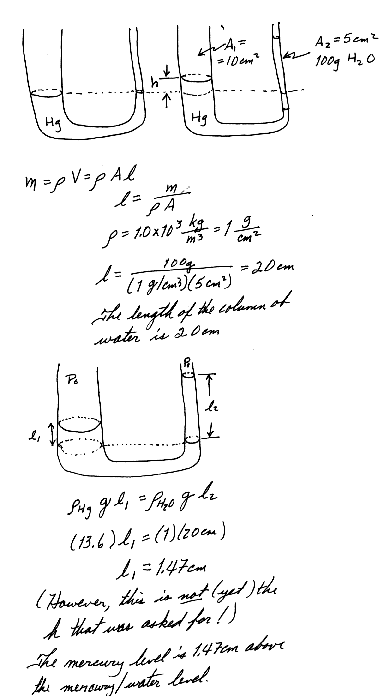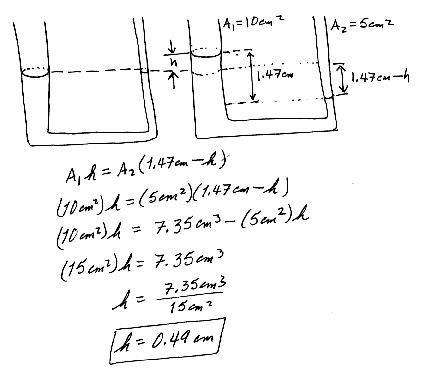15.16 Normal atmospheric pressure is 1.013 x 105 Pa. The approach of a storm causes the height of a mercury barometer to drop by 20 mm from the normal height. What is the atmospheric pressure? The density of mercury is 13.59 g/cm3.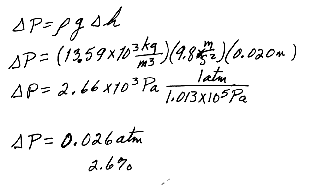15.38 Old Faithful Geyser in Yellowstone Nat'l Park erupts at approximately 1-hour intervals and the height of the fountain reaches 40 m.

(a) With what speed does the water leave the ground?

(b) What is the pressure (above atmospheric) in the heated underground chamber if its depth is 175 m?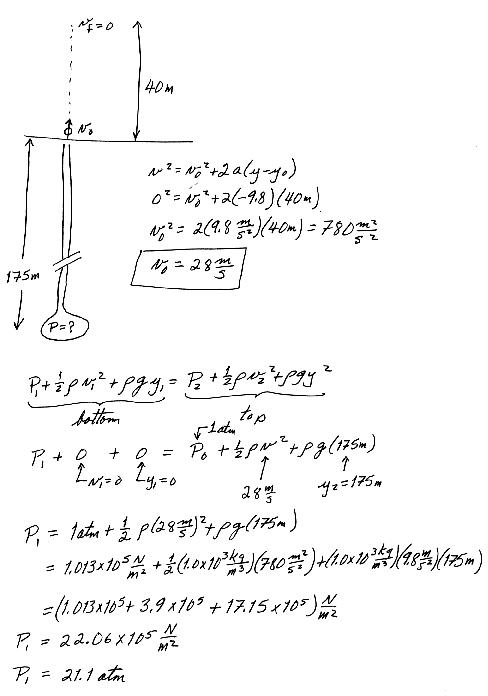15.41 A Pitot tube can be used to determine the velocity of air flow by measuring the difference between the total pressure and the static pressure (Fig P15.41). If the fluid in the tube is mercury, densityHg = 13.6 kg/m3m and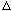h = 5.0cm, find the speed of air flow. Assume that the air is stagnant at point A and takeair = 1.25 kg/m3.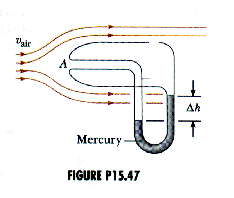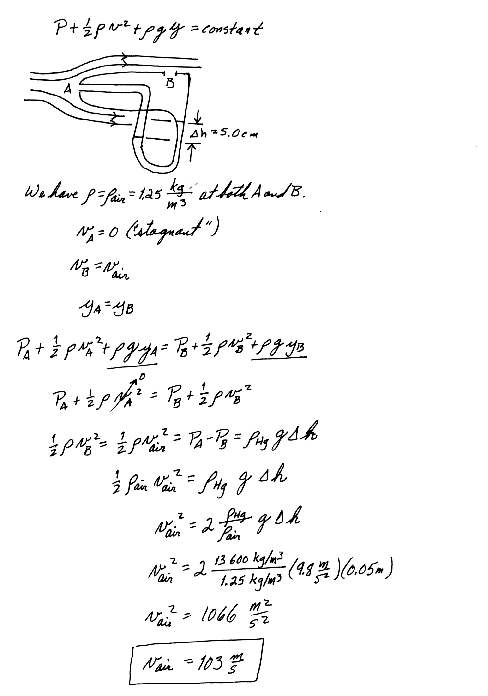15.46 A hole is punched in the side of a container of height ho, full of water as shown in Figure P15.49. If the water is to shoot as far as possible horizontally,

(a) how far from the bottom of the container should the hole be punched?

(b) Neglecting friction losses, how far (initially) from the side of the container will the water land?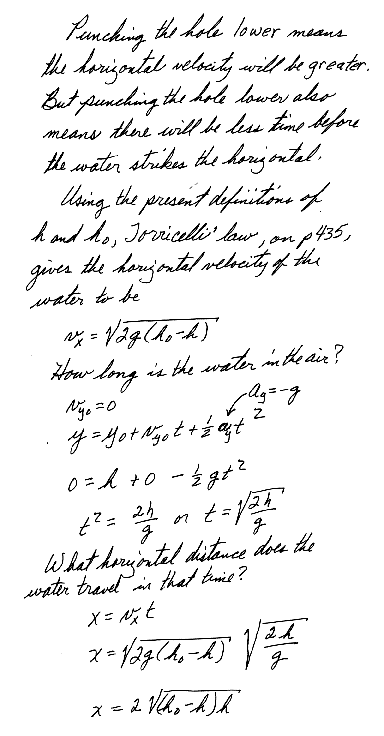Solutions to the additional problems from Serway's fourth edition

(4 ed) 15.1 A king orders a gold crown having a mass of 0.5 kg. When it arrives from the metalsmith, the volume of the crown is found to be 185 cm3. Is the crown made of solid gold?(4 ed) 15.2 Determine the absolute pressure at the bottom of a lake that is 30 m deep.(4 ed) 15.3 A simple U-tube that is open at both ends is partially filled with water (Fig. P(4)15.3). Keroseen of densityk is then poured into one arm of the tube, forming a column of height hk, as shown in the diagram. What is the difference h in the heights of the two liquid surfaces?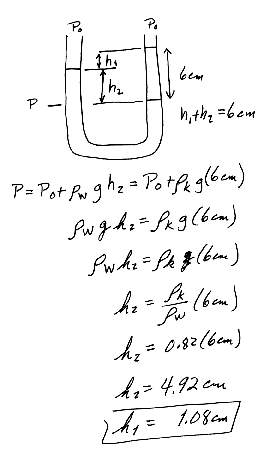(4 ed) 15.4 The rate of flow of water through a horizontal pipe is 2.00 m3/min. Determine the speed of flow at a point where the diameter of the pipe is

(a) 10.0 cm, and

(b) 5.0 cm.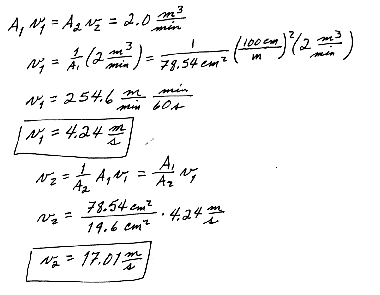(4 ed) 15.5 The Garfield Thomas water tunnel at Pennsylvania State University has a circular cross-section that constricts from a diameter of 3.6 m to the test section which is 1.2 m in diameter. If the speed of flow is 3.0 m/s in the larger-diameter pipe, determine the speed of flow in the test section.